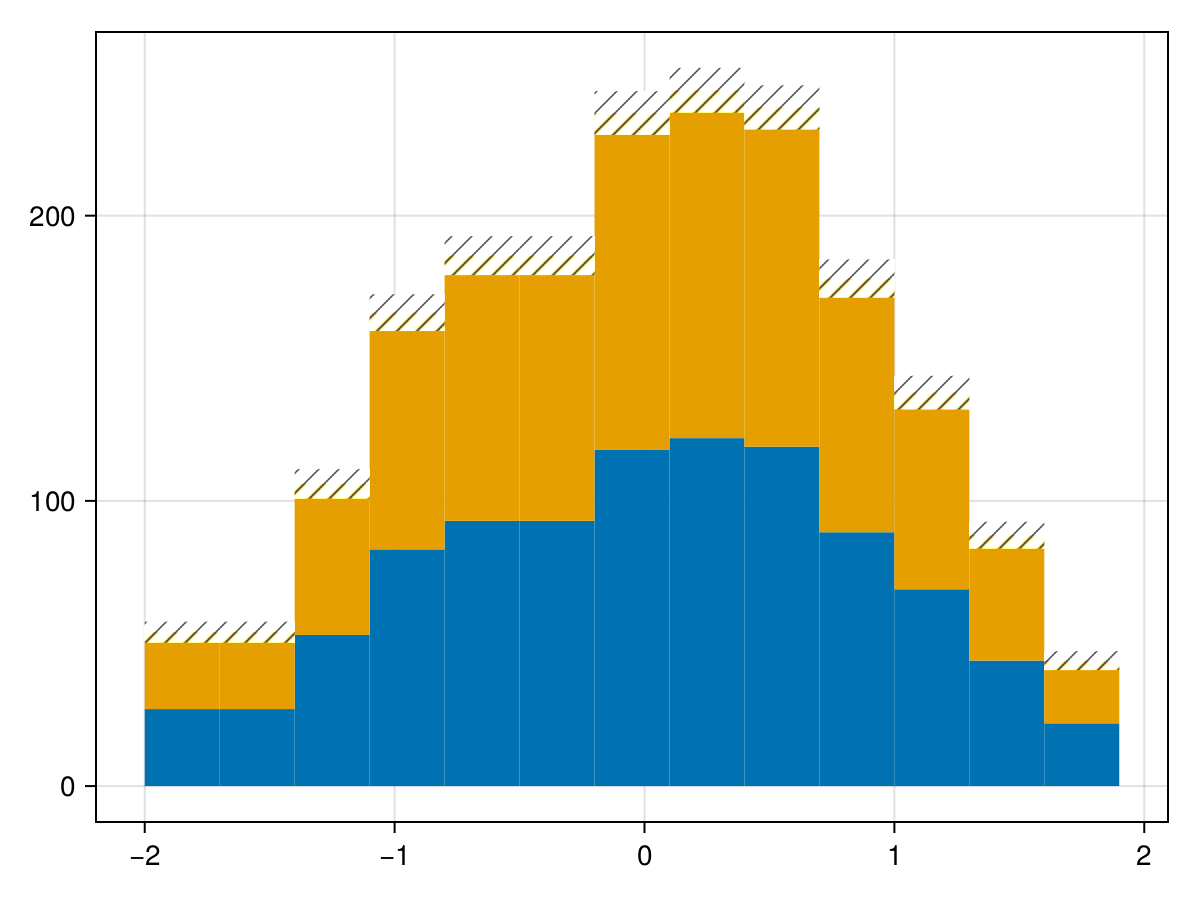using FHist, CairoMakie

Let's generate three dummy histograms sampled from three different distributions:

1. unit Gaussian with 1000 points

2. unit Gaussian with 10000 points

3. unit Gaussians with 1000 points and a mean of 0.5

begin
h1 = Hist1D(randn(10^3), -2:0.3:2)
h2 = Hist1D(randn(10^4), -2:0.3:2)
h3 = Hist1D(randn(10^3) .+ 0.5, -2:0.3:2)
end
• edges: -2.0:0.3:1.9
• bin counts: [9, 13, 30, 39, 60, 83, 101, 118, 113, 128, 82, 65, 62]
• maximum count: 128
• total count: 903

To plot a single histogram, you can do any of the following:

begin
fig = Figure()
p1, ax1= plot(fig[1, 1],h1; label = "plot(h1)")
p2, ax2 = stairs(fig[1, 2], h1, label = "stairs(h1)")
p3, ax3 = hist(fig[2, 1], h1, label = "hist(h1)")
p4, ax4 = errorbars(fig[2, 2], h1, color=:black, whiskerwidth=6, label = "errorbars(h1)")
axislegend.([p1, p2, p3, p4])
fig
end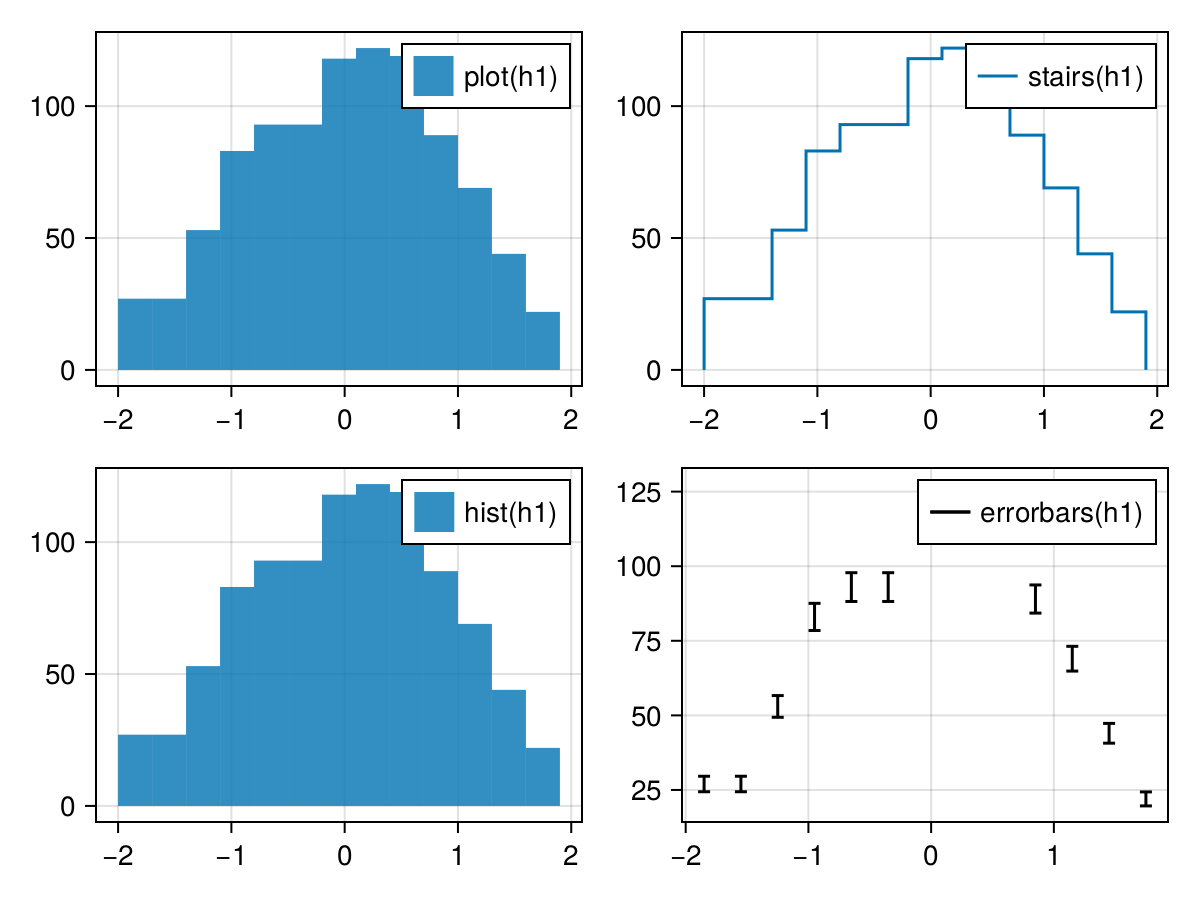When you're studying a single histogram, it's hepful to have statbox (CERN ROOT enjoyer?)

We provide a statbox!() function to add such box:

begin
f_s = stairs(h1)
statbox!(f_s, h1)
f_s
end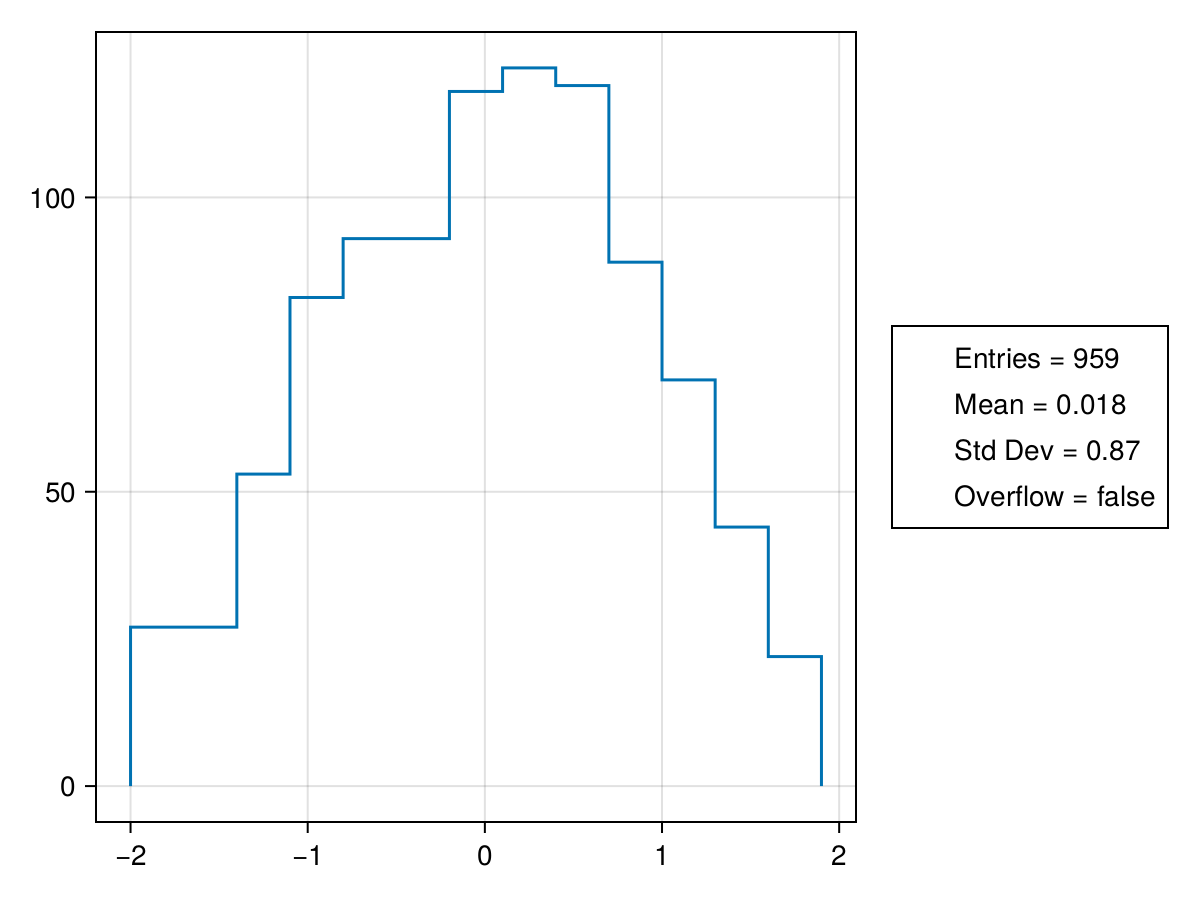statbox also works for 2D histogram as one'd expect:

begin
h2d = Hist2D((randn(10000), randn(10000)))
p2d = plot(h2d)
statbox!(p2d, h2d)
p2d
end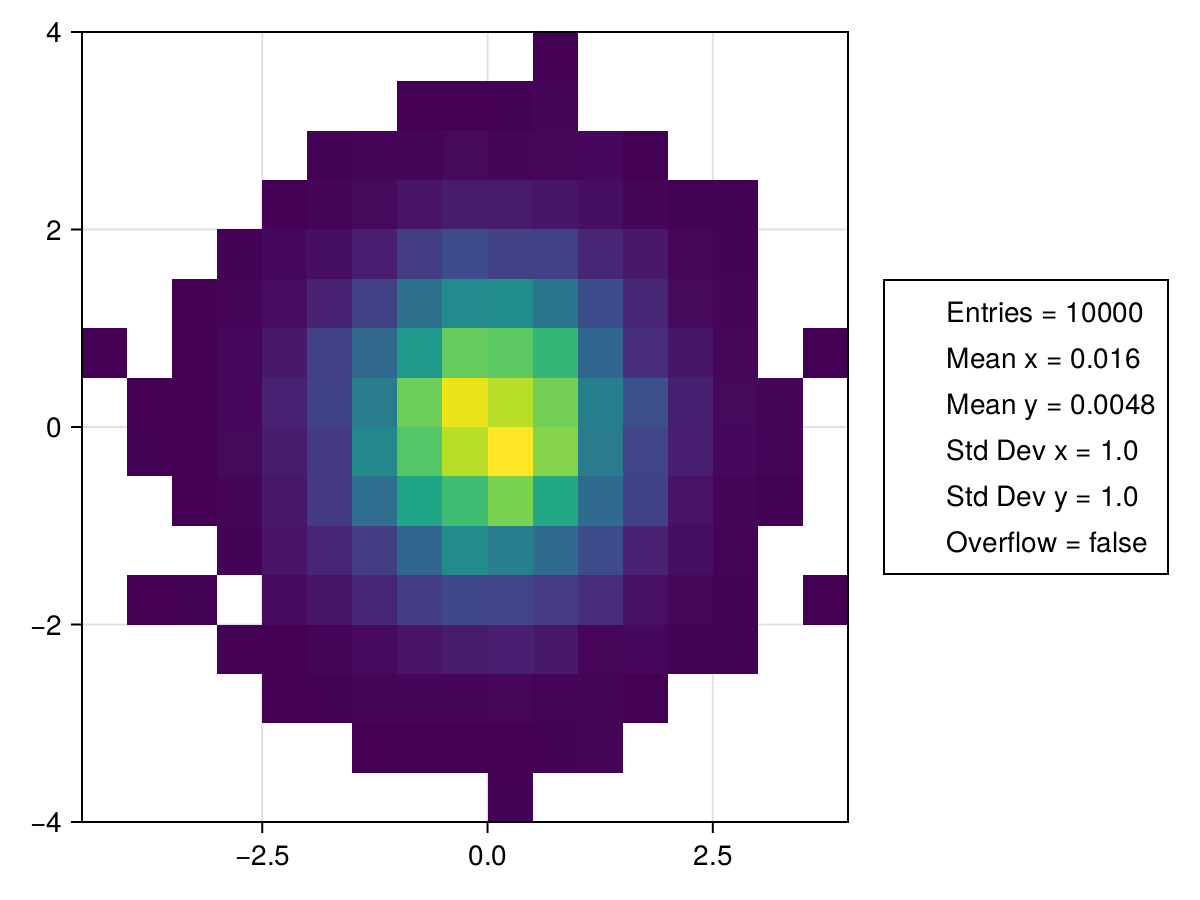You can freely combine these by using the bang ! version to plot things on top of each other, for example this is some plot you might see: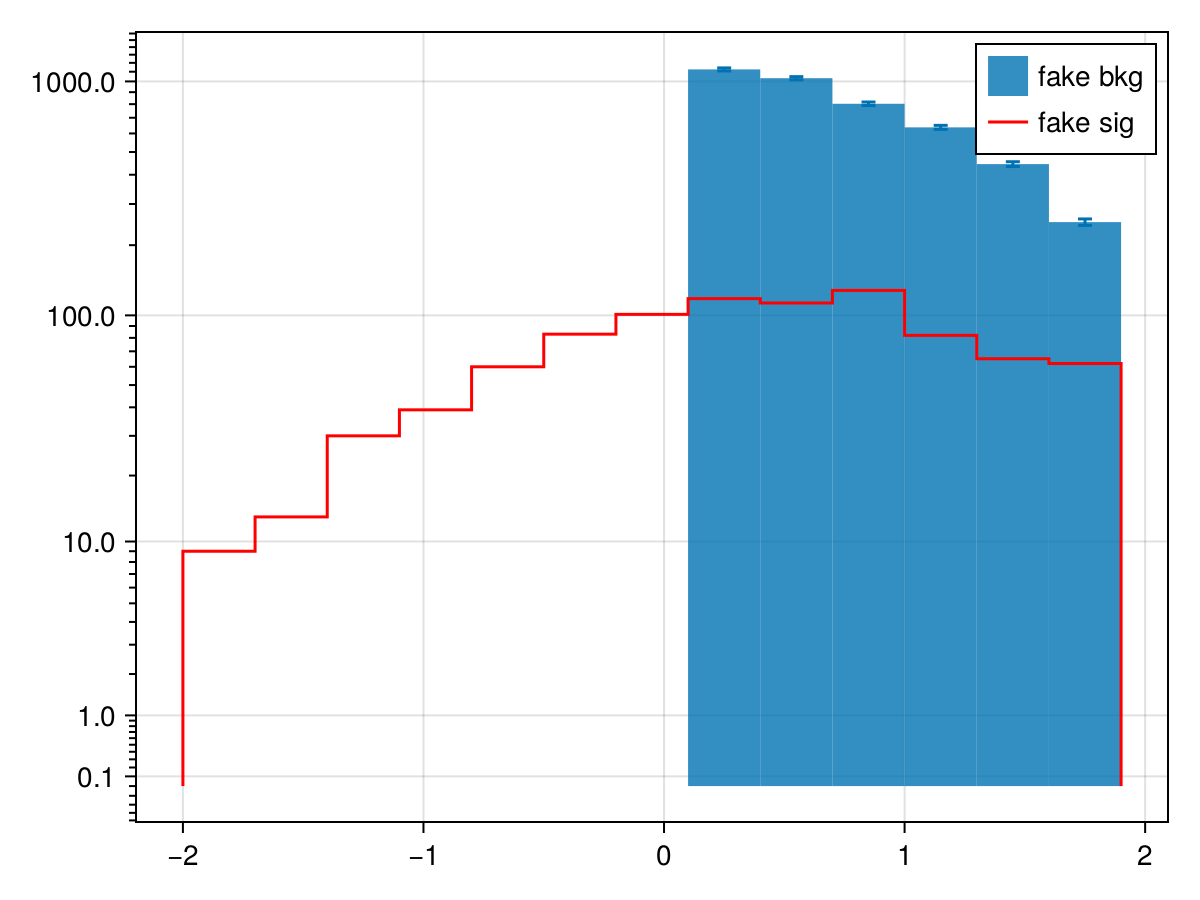But it's also pretty common to have multiple background components (processes), in such case, we often want a stacked histogram with error bar representing the sum of each component added up correctly.

We also have a recipe for that:

with_theme(ATLASTHEME) do
f, a, p = stackedhist([h2, h2, h1 ])
labels = ["proc1", "blah", "third one"]
elements = [PolyElement(polycolor = p.attributes.color[][i]) for i in 1:length(labels)]
title = "Legend title"
Legend(f[1,2], elements, labels, title)
f
end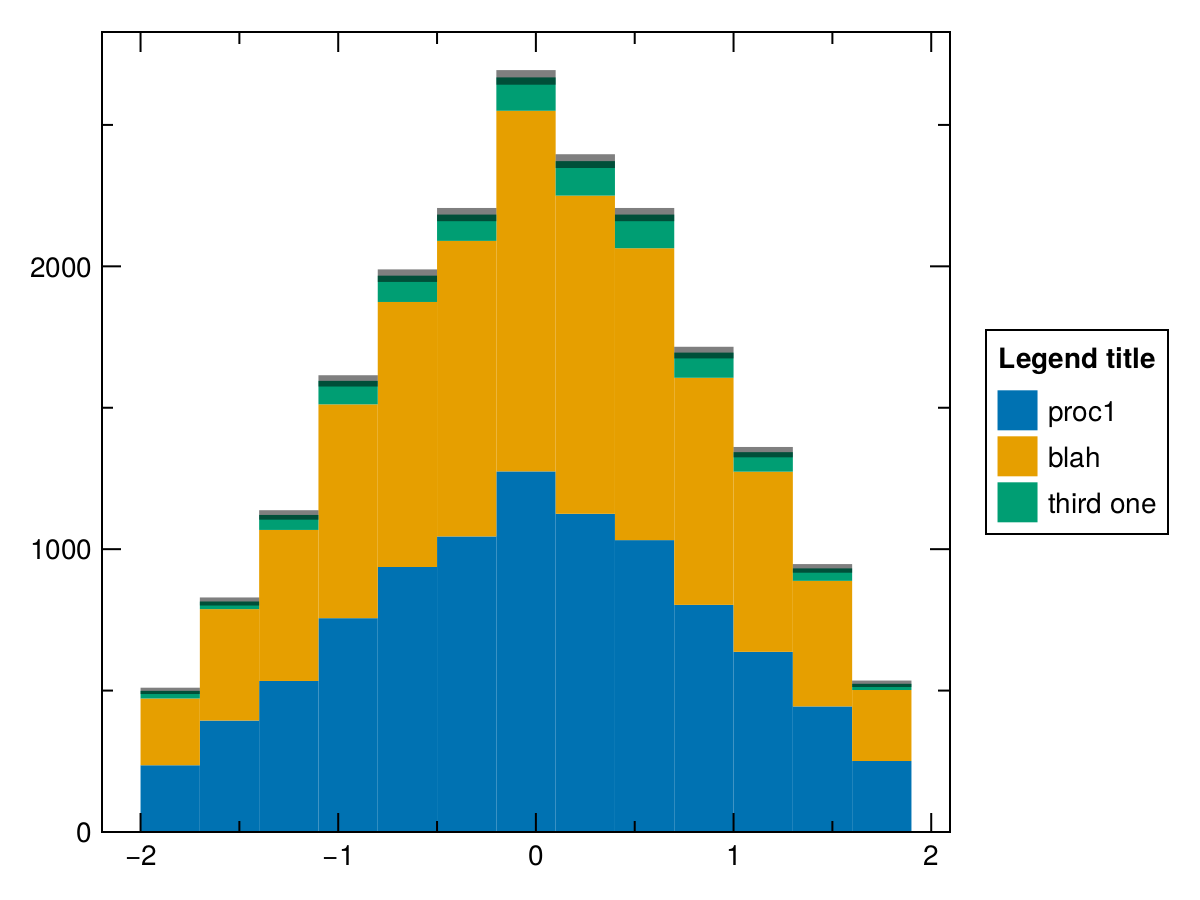Notice the with_theme(ATLASTHEME), you can apply a bunch of appearance tweaks for the entire plot.

Finally, we have a recipe for ratio plot which is argubaly useful for the bottom pannel in many plots:

with_theme(ATLASTHEME) do
f_ratio, a, p = stairs(h1; label="h1")
errorbars!(a, h1)
stairs!(a, h3; label="h3")
errorbars!(a, h3)

axislegend()
ratioax = Axis(f_ratio[2, 1], aspect = 5.5, xlabel = "my x-axis", ylabel="h1 / h3")
hidexdecorations!(a)
ratiohist!(ratioax, h1/h3; color=:black, errors=true)
rowsize!(f_ratio.layout, 1, Aspect(1, 0.5))
f_ratio
end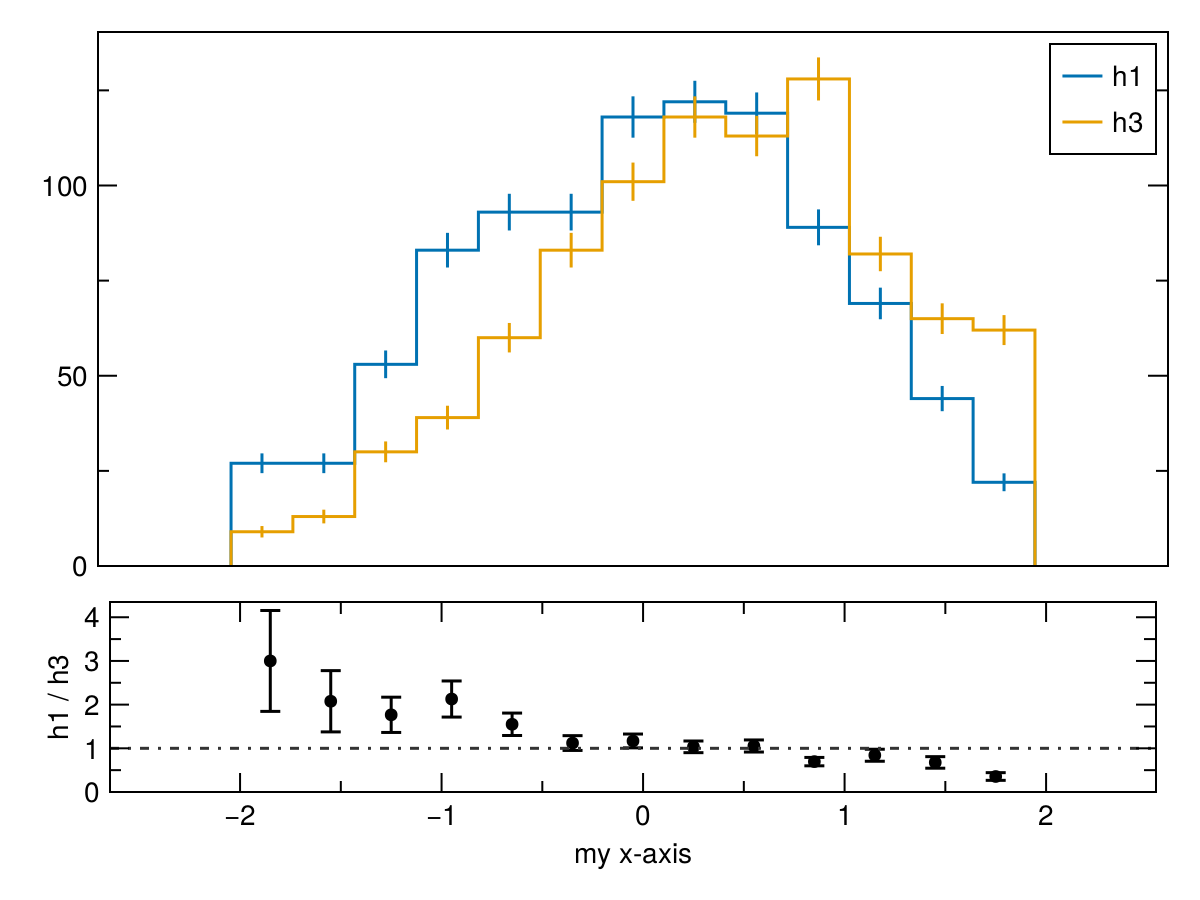Until: https://github.com/JuliaPlots/Makie.jl/issues/1385#issuecomment-1166367423 is fixed, we don't have access to LinePattern driven shading, so you'd have to be creative and use crossbar:

begin
f_crossbar = stairs(h1)
statbox!(f_crossbar, h1)
crossbar!(
h1;
show_midline=false,
color=(:black, 0.5),
gap=0, width=diff(binedges(h1))
)
f_crossbar
end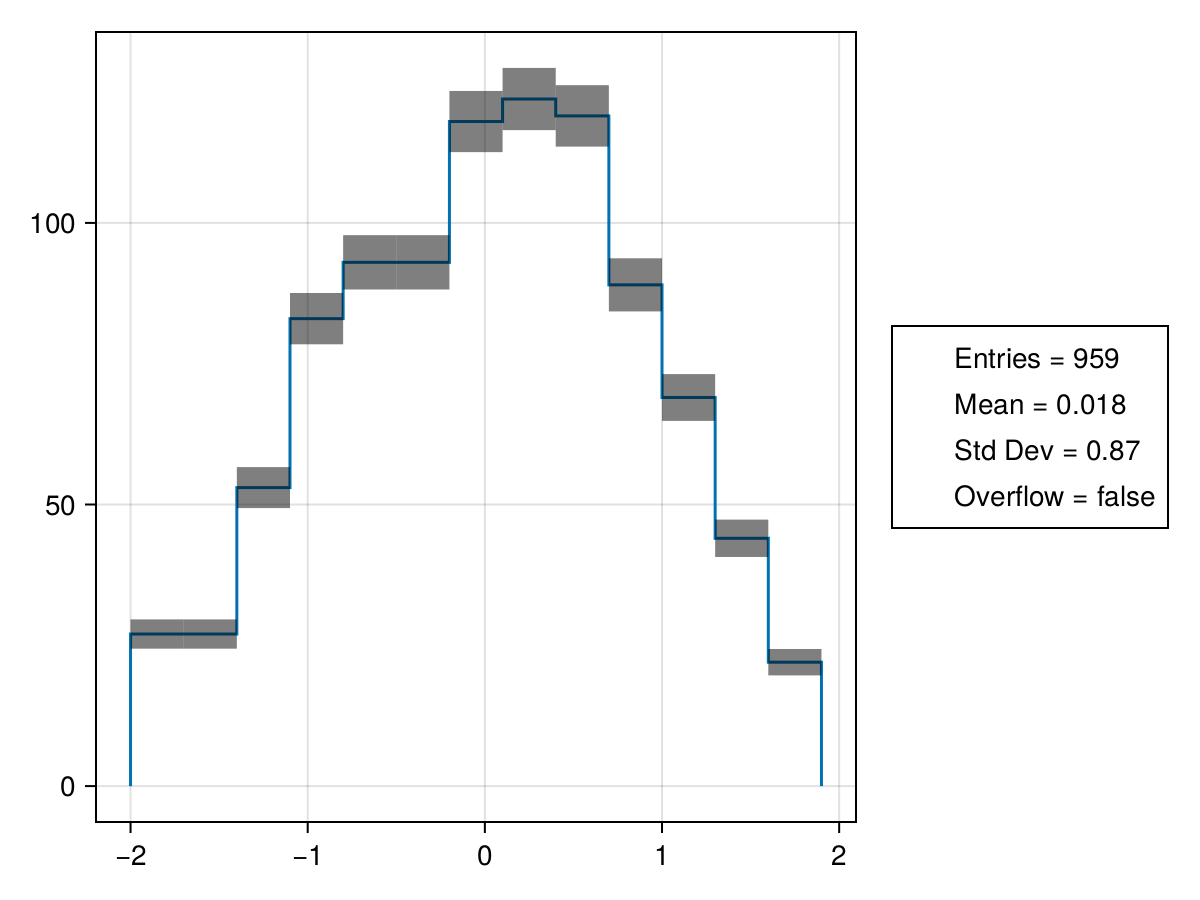let
f, a, p = stackedhist([h1, h1 ]; error_color=(:black, 0.5))
labels = ["proc1", "blah"]
elements = [PolyElement(polycolor = p.attributes.color[][i]) for i in 1:length(labels)]
title = "Legend title"
Legend(f[1,2], elements, labels, title)
f
end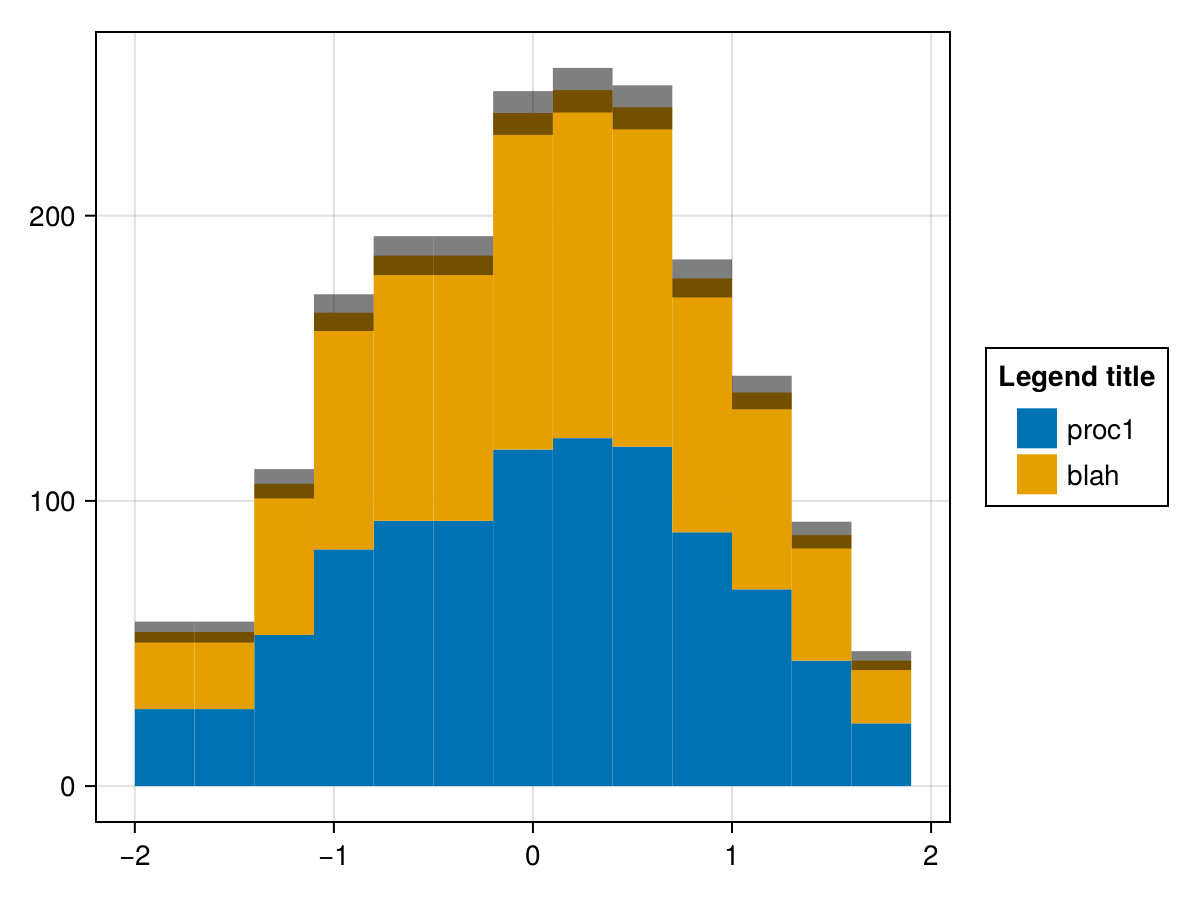let
f, a, p = stackedhist([h1, h1 ]; error_color=Pattern('/'))
f
end